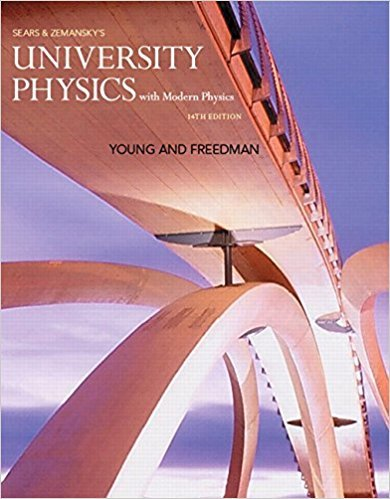×
×

# You run due east at a constant speed of 3.00 m>s for aISBN: 9780321973610 228

## Solution for problem Q2.20 Chapter 2

University Physics with Modern Physics (1) | 14th Edition

• Textbook Solutions
• 2901 Step-by-step solutions solved by professors and subject experts
• Get 24/7 help from StudySoup virtual teaching assistantsUniversity Physics with Modern Physics (1) | 14th Edition

4 5 1 260 Reviews
27
3
Problem Q2.20

You run due east at a constant speed of 3.00 m>s for a distance of 120.0 m and then continue running east at a constant speed of 5.00 m>s for another 120.0 m. For the total 240.0-m run, is your average velocity 4.00 m>s, greater than 4.00 m>s, or less than 4.00 m>s? Explain.

Step-by-Step Solution:
Step 1 of 3

1. Transforming energy A. Energy is not lost it is just converted to less important forms B. Work into a system: positive C. Work out of a system: negative D. Work includes electric energy into and out of motors and generators E. Energy output= energy input F. Thermal energy is...

Step 2 of 3

Step 3 of 3

##### ISBN: 9780321973610

This textbook survival guide was created for the textbook: University Physics with Modern Physics (1), edition: 14. This full solution covers the following key subjects: . This expansive textbook survival guide covers 44 chapters, and 4574 solutions. University Physics with Modern Physics (1) was written by and is associated to the ISBN: 9780321973610. The full step-by-step solution to problem: Q2.20 from chapter: 2 was answered by , our top Physics solution expert on 01/09/18, 07:46PM. Since the solution to Q2.20 from 2 chapter was answered, more than 229 students have viewed the full step-by-step answer. The answer to “You run due east at a constant speed of 3.00 m>s for a distance of 120.0 m and then continue running east at a constant speed of 5.00 m>s for another 120.0 m. For the total 240.0-m run, is your average velocity 4.00 m>s, greater than 4.00 m>s, or less than 4.00 m>s? Explain.” is broken down into a number of easy to follow steps, and 54 words.

Unlock Textbook Solution